# CBSE Physics | Solved Numerical on Force and Laws of Motion

## Solved Numerical on Force and Laws of Motion

### Numerical- Solving Tips

Step 1 : Read the question carefully and visualise the situation (think as if the process is happening in front of you).

Step 2 : Draw a relevant figure and write the parameters given.

Step 3 : Make sure all values are in the same set of units.

Step 4 : Plan the concept/ formulae which are to be used.

Step 5 : Avoid doing calculations in each step. Try to do all the calculations at final step to save time.

### Question 1. A motorcycle of mass of 2000 kg is moving over a horizontal road, with uniform velocity. If this motorcycle has to be stopped with a negative acceleration of 1.5 ms–2, then what is the force of friction between the types of the motorcycle and the road?

Solution:

Here, m = 2000 kg; a = – 1.5 ms–2; F = ?

Using, F = ma, we get

F = 2000 kg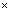(– 1.5) ms –2 = – 3000 kg ms– 2

= – 3000 N

Negative sign shows that force of friction (F) acts in a direction opposite to the motion of the motorcycle.

### Question 2. A constant force acts on an object of mass 5 kg for a duration of 2 s. It increases the object’s velocity from 3 ms–1 to 7 ms–1. Find the magnitude of the applied force. Now, if the force was applied for a duration of 5 s, what would be the final velocity of the object?

Solution:

Given u = 3 ms–1, v = 7 ms–1, t = 2s, m = 5 kg

Also F = ma

u = 3 ms–1, t = 5 s, a = 2 ms– 2, v = ?

Applying, v = u + at

### Question 3. A bus of mass 5000 kg starts from rest and rolls down a hill. If it travels a distance of 200 m in 10s. Calculate (i) acceleration of the bus and (ii) the force acting on the bus.

Solution:

Here, mass m = 5000 kg; u = 0; s = 200 m; t = 10 s;

a = ?; F = ?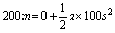or a = 4 ms – 2.

Using , F = ma, we get

F = 5000 kg4 ms– 2 = 20,000 kg ms– 2

= 20,000 N

### Question 4. The velocity-time graph of a ball m moving on the surface of floor is shown in figure below. Calculate the force acting on the ball, if mass of the ball is 20 g.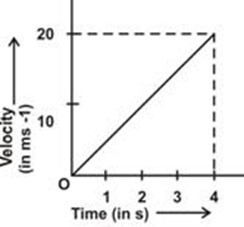Solution:

The velocity-time graph shows that the velocity of the ball at t = 0 is zero. That is initial velocity of ball, u = 0

Velocity of ball at t = 4 s is 20 ms– 1

That is, final velocity, v = 20 ms – 1

time, t = 4 s

a = ? ; F = ?

Or a = 5 ms– 2

= 0.1 N

### 5. The velocity-time graph of a ball moving on the surface of a floor is shown in fig below. Find the force acting on the ball if the mass of the ball is 50 g.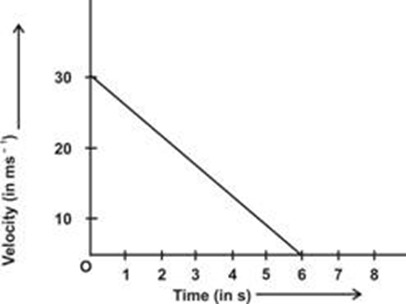Solution:

The velocity-time graph shows that velocity of the ball at t = 0 is 30 ms–1

That is, initial velocity of the ball, u = 30 ms–1

The velocity of the ball at t = 6s is zero.

That is, final velocity of the bal,= 0

time, t = 6s ; F = ?

= – 5 ms–2

Negative sign shows that the ball is retarded or decelerated.

Step 2. Also, mass of ball, m = 50 g### Question 6. An iron sphere of 1 kg is moving a velocity of 20 ms– 1 on a cemented floor. It comes to rest after traveling a distance of 50 m. Find the force of friction between the sphere and the floor.

Solution.

Here, m = 1 kg.; u = 20 ms – 1;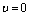; s = 50 m; F = ?

= – 4 ms– 2

Step 2. Using F = ma, we get

= – 4 kg ms– 2 = – 4N

Negative sign shows that the force is retarding force.

### Question 7. A wooden block of mass m1 kg accelerates at 10 ms–2 when a force of 5 Newton acts on it. Another block of mass m2 kg accelerates at 20 ms–2 when the same force acts on it. Find the acceleration, if both the blocks are tied together and same force acts on this combination.

Solution.

For First Block: m = m1 kg.; a = 10 m/s2

Using F = ma, we get

For Second Block:

m = m2 kg; a = 20 ms– 2; F = 5 N

Using, F = ma, we get

When both the blocks are tied together

Mass of the combination, m = (m1 + m2) = ( 0.5 kg + 0.25 kg) = 0.75 kg

F = 5 N

Using F = ma, we get

### Question 8. A car of mass 1000 kg moving with a velocity of 36 km/h hits a wall and comes to rest in 5 s. Find the force exerted by the car on the wall.

Solution.

Here, m = 1000 kg

Time, t = 5 s.

F = ?

Using, F = ma, we get

Thus force exerted by the car on the wall = 2000N

### Question 9. A ball of mass 100 g moving with velocity of 10 ms – 1 is stopped by a boy in 0.2s. Calculate the force applied by the boy to stop the ball.

Solution.

Here, mass of ball, m = 100g =kg = 0.1 kg

Initial velocity of ball, u = 10 ms–1

Time, t = 0.2 s.

F = ?

step 2. Using, F = ma, we get

F = 0.1 kg ( – 50 ms– 2) = – 5 kg ms– 2 = – 5 N

Negative sign shows that force applied by the boy is the opposing force i.e. a force in a direction opposite to the direction of motion of the ball.

### Question 10. A car of mass 1000 kg and bus of mass 8000 kg are moving with same velocity of 36 kmh– 1. Find the forces to stop both the car and the bus in 5 s.

Solution.

Here, initial velocity, u = 36 km h–1 =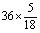ms–1= 10 ms–1

Time t = 5 s.

Mass of car, m = 1000 kg

F = ma = 1000 kg (– 2 ms – 2) = – 2000 N

Setp 2. Mass of the bus, m = 8000 kg

F = ma = 8000 kg (– 2 ms– 2) = – 16000 N

Negative sign with force shows that the force is applied in a direction opposite to the direction of the motion of the car and bus. Such a force is known as retarding force.

### Question 11. A body of mass 100 g is at rest on a smooth surface. A force of 0.2 newton acts on it for 5 seconds. Calculate the distance traveled by the body.

Solution.

F = 0.2 N

t = 5 s

u = 0

S = ?

Step 1. F = ma, we get

Step 2. To find distance traveled, using

### Question 12. A gun fires a bullet of mass 50g. The bullet moving with a velocity of 100 ms– 1 strikes a wooden plank and comes to rest in 0.05 s. Calculate (i) the force exerted by the wooden plank on the bullet and (ii) the distance of penetration of the bullet in the wooden plank.

Solution.

u = 100 ms – 1

t = 0.05 sec

F = ? ; S = ?

(i) Using, F = ma, we get

= – 100 kg ms – 2 = –100 N

Thus, force exerted by the wooden plank on the bullet = 100 N.

= 5 m – 2.5 m = 2.5 m.

### Question 13. A mechanic strikes a nail with a hammer of mass 500 moving with a velocity of 20 ms – 1.The hammer comes to rest in 0.02 s after striking the nail. Calculate the force exerted by the nail on the hammer.

Solution.

Using, F = ma

Therefore, force exerted by nail on the hammer = 500 N

### Question 14. A bullet of mass 100 g is fired from a gun of mass 20 kg with a velocity of 100 ms– 1. Calculate the velocity of recoil of the gun.

Solution.

Mass of gun, M = 20 kg

Let recoil velocity of gun = V

Step 1.Before firing, the system (gun + bullet) is at rest therefore, initial momentum of the system = 0

Final momentum of the system = momentum of bullet + momentum of gun

Step 2. Apply law of conservation of momentum

Final momentum = Initial momentum

i.e. 10 + 20 V = 0

20 V = – 10

Negative sign shows that the direction of recoil velocity of the gun is opposite to the direction of the velocity of the bullet.

### Question 15. An iron sphere of mass 10 kg is dropped from a height of 80 cm. If the downward acceleration of the ball is 10 ms– 2, calculate the momentum transferred to the ground by the ball.

Solution.

Here, Initial velocity of sphere, u = 0

Distance travels, S = 80 cm = 0.8 m

Acceleration of sphere, a = 10 ms – 2

Step 1. Final velocity of sphere when it just reaches the ground can be calculated using

= 16 m2 s– 2

= 4 ms–1

Momentum of the sphere just before it touches the ground =Step 2. On reaching the ground, the iron sphere comes to rest, so its final momentum = 0

According to the law of conservation of momentum,

Momentum transferred to the ground = momentum of the sphere just before it comes to rest

= 40 kg ms– 1

### Question 16. Two small glass spheres of masses 10 g and 20 g are moving in a straight line in the same direction with velocities of 3 ms – 1 and 2 ms–1 respectively. They collide with each other and after collision, glass sphere of mass 10g moves with a velocity of 2.5 ms– 1. Find the velocity of the second ball after collision.

Solution.

u1 = 3 ms–1; u2 = 2 ms– 1

Momentum of first sphere before collision = m1u1

Momentum of second sphere before collision = m2u2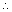Total momentum of both the sphere before collision = m1u1 + m2u2

Now, momentum of first sphere after collision =Momentum of second sphere after collision =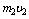Total momentum of both the spheres after collision

Now, according to the law of conservation of momentum.

Total momentum after collision = Total momentum before collision

= 2.25 ms–1

### Question 17. Two bodies each of mass 0.5 kg are moving in a straight line but opposite in direction with the same velocity of 2 ms– 1. They collide with each other and stick to each other after collision. What is the common velocity of these bodies after collision?

Solution.

Let one body is moving towards left side and second body is moving to the right side. So velocity of the body to the left side is taken as positive and velocity of body moving to the right side is taken as negative.

Momentum of body moving to left side before collision = mv

Momentum of body moving to right side before collision = – mv

= – 1 kg ms–1Total momentum of both the bodies before collision

= 1 kg ms–1 – 1 kg ms–1 = 0

Let V = common velocity of both the bodies after striking to each otherTotal momentum of both the bodies after collision

= (m1 + m2) V

= (0.5 + 0.5) V = V kg ms– 1

According to the law of conservation of momentum

Total momentum after collision = Total momentum before collision

i.e., V = 0

Thus, the combination of two bodies comes to rest after collision.

This site uses Akismet to reduce spam. Learn how your comment data is processed.